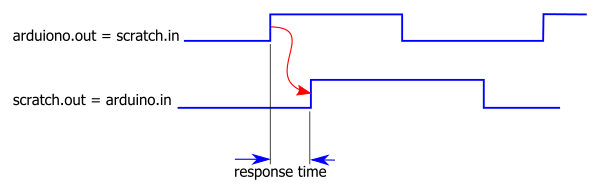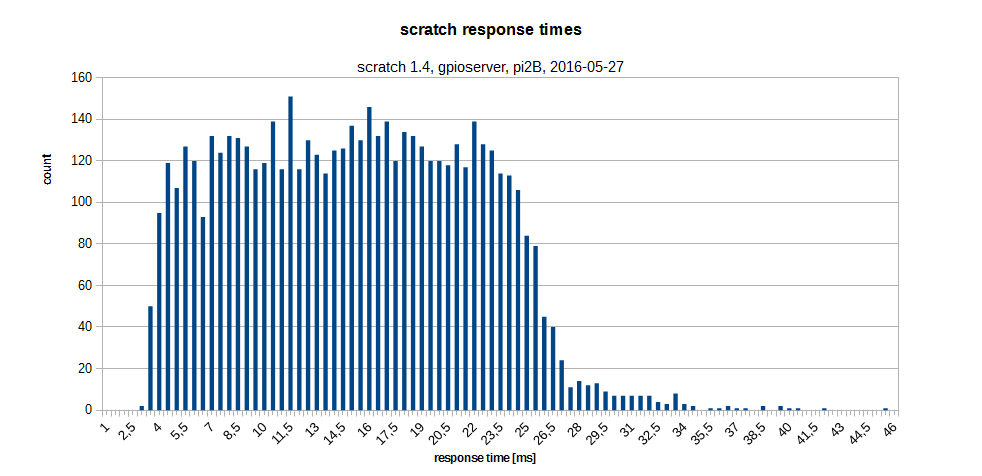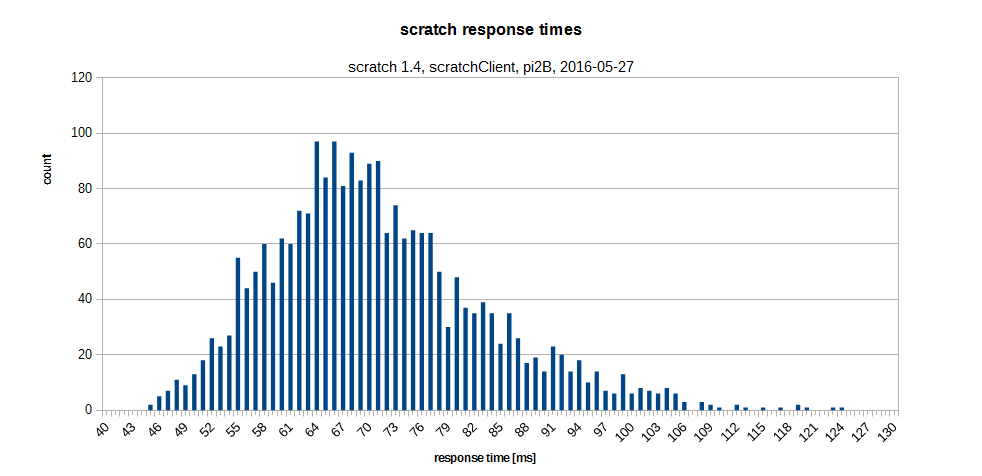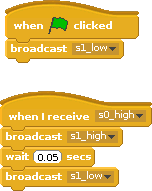# scratch io response times

Scratch 1.4 on raspberry pi allows programs to work with GPIO pins. I was curious to see how fast scratch can react to input values.

To measure these times, I connected an arduino due (84MHz clock) with a raspberry pi 2B. The arduino produces a 0–>1 edge for scratch and scratch responds with a 0–>1-edge on another pin. The red arrow in the chart shows this dependency. The time between these two edges is the response time of scratch.The scratch code is straightforward. Sensor values are 0, 1, so there are logical operations needed.

Response times are aggregated in a chart. X-axes shows the time intervals, y-axis shows how often execution times have been measured in the 0.5 ms intervals.The response times range from  3ms to 46ms. The bulk of values is between 5ms and 27ms. The chart is produced from 5659 single measurements. So scratch is sufficient fast to respond in slow environments, but should not be used to control fast systems.

Here the arduino code:

```//
// run on an arduino due

int pout = 11;
int pin = 12;

void setup() {
Serial.begin(9600);
while (!Serial) {
; // wait for serial port to connect. Needed for native USB port only
}
Serial.println("response_time_measurement");
pinMode(pin, INPUT);
pinMode(pout, OUTPUT);
digitalWrite(pout, LOW);
}
long randomnumber = 0;
long t0 = 0;
long t1 = 0;

void loop() {
// wait for input low
while ( HIGH == digitalRead(pin)) ;

randomnumber = random(1, 700);
delay(randomnumber);
t0 = micros();
digitalWrite(pout, HIGH);

while ( LOW == digitalRead(pin)) ;
t1 = micros();

Serial.print(randomnumber);
Serial.print("\t");
Serial.println(t1 - t0);

delay(100);
digitalWrite(pout, LOW);
}
```

There is a variable delay in the arduino code from 1 to 700ms to prevent a ‘lock in’ to scratch cycle times.

Although time measurements are in microseconds, the accuracy depends on how good the compiler is in producing fast code. As the times to be measured are in millisecond range, this approach is reasonable precise.

Similiar setup with scratchClient yields following results:Times are centered around some 67 ms, min is 46 ms which is slower than using gpioserver.

The scratchClient-adapter used is ‘GpioButtonInput’, which already provides edge-detection. The scratch code is